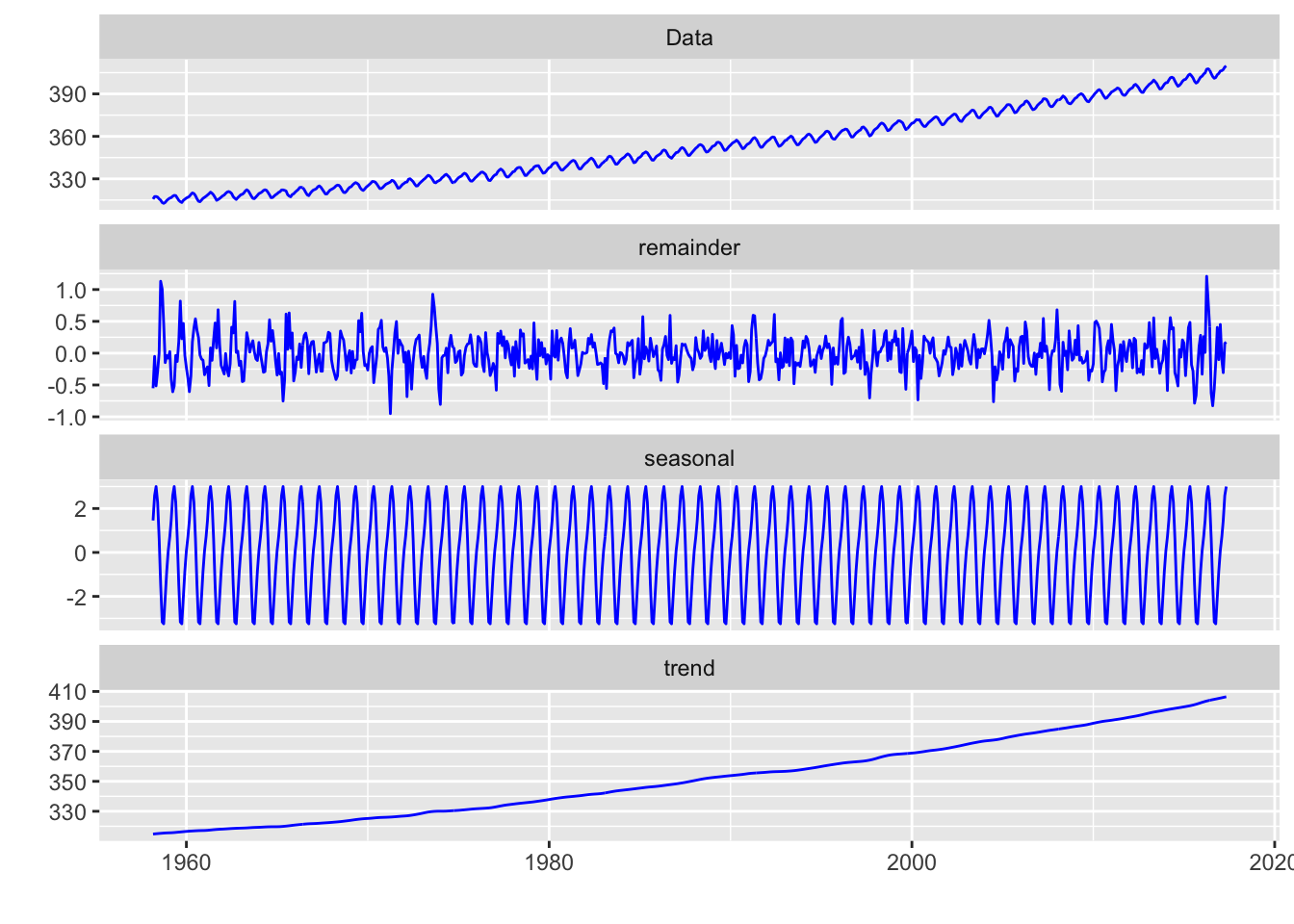STL is an acronym for Seasonal and Trend decomposition using Loess, while loess (locally weighted regression and scatterplot smoothing) is a method for estimating nonlinear relationships.

We start our analysis with the creation of a `ts` object. This is done by the `ts()` function. We make use of the `first` and `last` function from the `xts` package and the `year` and `month` function from the `lubridate` package to extract the starting year/month (`start`) and the ending year/month (`end`) combinations of the data set programmatically.

``````library(xts)

library(lubridate)
start <- c(year(xts::first(co2)), month(xts::first(co2)))
start``````
``##  1958    3``
``````end <- c(year(xts::last(co2)), month(xts::last(co2)))
end``````
``##  2017    5``
``````# creation of a ts object
co2 <- ts(data = as.vector(coredata(co2)),
start = start,
end = end, frequency = 12)``````

In R the `stl()` function performs decomposition of a time series into seasonal, trend and irregular components using Loess.

The function requires the `s.window` argument, which is either the character string `"periodic"` or the span (in lags), an odd number, of the loess window for seasonal extraction. The default value is 7. Another requested argument is `t.window`, which is the span (in lags, not absolute time) of the loess window for trend extraction; this must also be odd. The argument has a default value related to the value `s.window`. Type `help(stl)` into your console for more information on the `stl()` function.

After we applied the `stl()` function we plot the result with the convenient `autoplot()` function.

``````# set up stl function
fit <- stl(co2, s.window = "periodic")

library(ggfortify)
autoplot(fit, ts.colour = 'blue')``````The plot shows four panels. In the uppermost we see the raw data, the Keeling curve. In the subsequent plot we see the trend component, the seasonal component and the remainder. We realize a very strong linear trend in the data set. Let us redo the analysis, however this time we focus on the 21st century.

``````co2.2000 <- window(co2,
start = c(2000,1),
end = c(2015,12))

fit.2000 <- stl(co2.2000,  s.window = "periodic")
autoplot(fit.2000, ts.colour = 'blue')``````